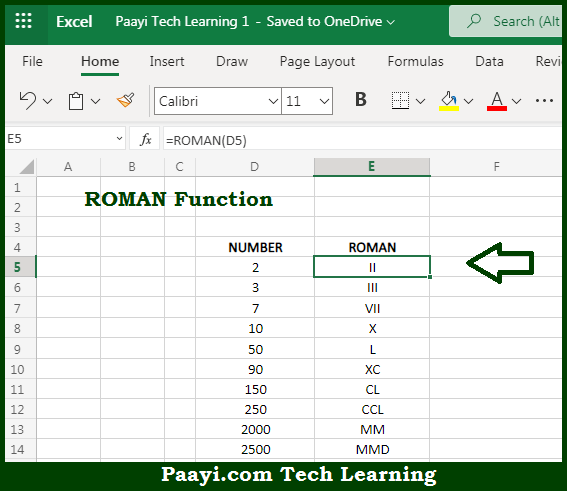# Learn How to Use Microsoft Excel ROMAN Function

Written by | 0 Comments | 581 Views

In this article, you will learn how to use the Microsoft Excel ROMAN function and its prime function in Microsoft Excel. You will also get to know the Microsoft Excel ROMAN function return value and syntax with the help of some examples.

Microsoft Excel ROMAN Function

The main function of the Microsoft Excel ROMAN function is to get the converted roman number from the given numbers. That implies, with the help of the ROMAN function you can able to return converted roman numbers as a text. So, with the help of the ROMAN function, you can able to convert the numbers to roman numerals as a text.

Return Value of ROMAN Function

The return value will be the roman numeral in text.

Syntax of ROMAN Function

=ROMAN(number, [form])

Where the arguments:

• bottom: This is the number in the Arabic numeral you want to convert into a roman numeral.
• top: This is the type of roman numeral you want to get (optional).

## How to Use Microsoft Excel ROMAN Function?So we know that Microsoft Excel ROMAN function you can able to get the converted roman number from the given numbers. That implies, with the help of the ROMAN function you can able to return converted roman numbers as text. It should be noted that the number must be positive and less than 3999. The roman function work opposite of the ARABIC function and the form argument controls whether the roman numeral can be abbreviated or not.

The argument is optional and the default to zero (classic non-abbreviated). The ROMAN function supports additional forms 1-4 which are progressively more concise. So, with the help of the ROMAN function, you can able to convert the numbers to roman numerals as a text.